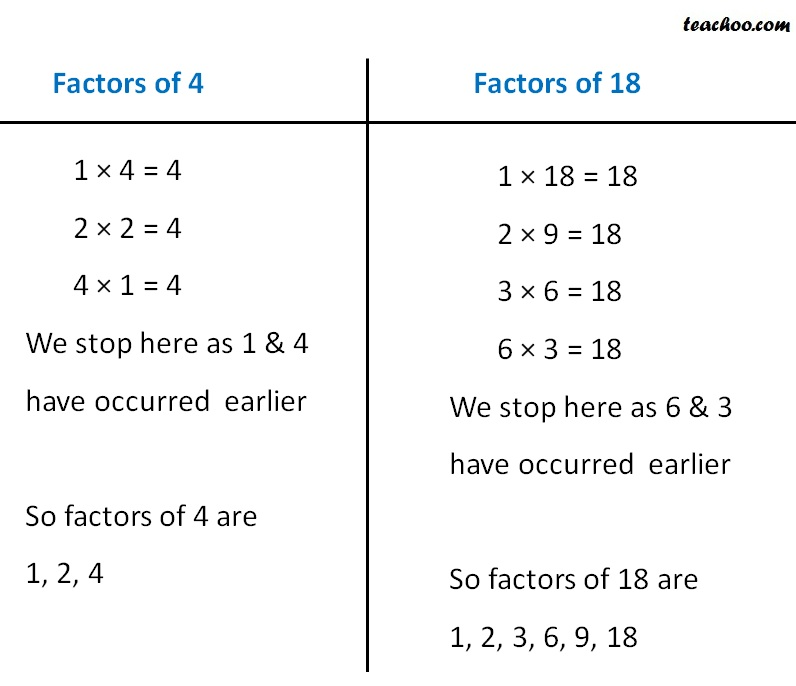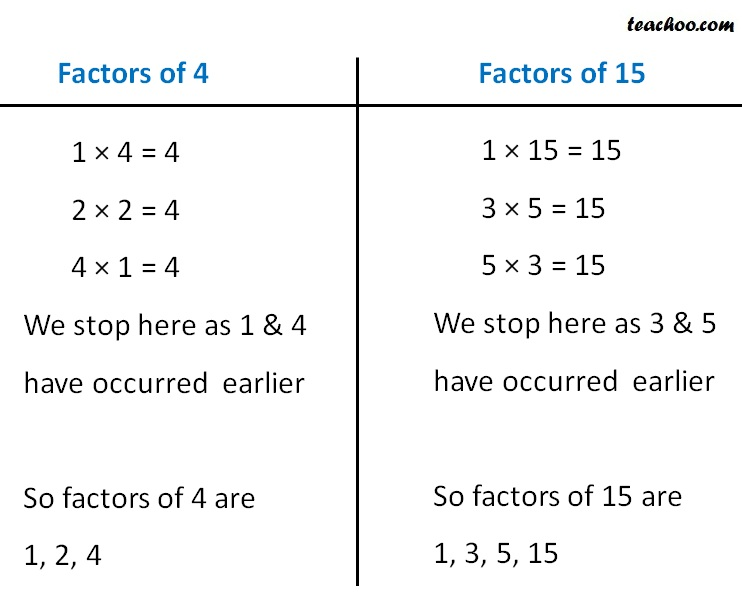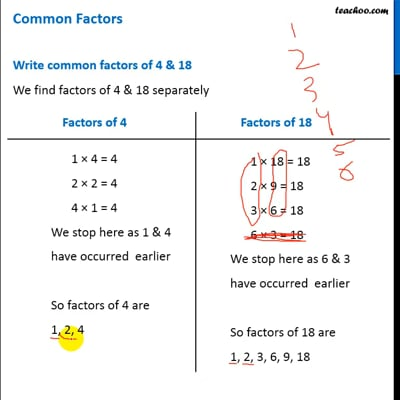Common Factors

Chapter 3 Class 6 Playing with Numbers
Concept wise

## Write common factors of 4 & 18

We find factors of 4 & 18 separatelySo, Common factors of 4 & 18 are 1, 2

## Write common  factors of 4 & 15

We find factors of 4 & 15 separatelySo, Common factors of 4 & 15 are 1

Note:

If two numbers have common factor 1

Then, they are co-prime numbersThis video is only available for Teachoo black users

### Transcript

Factors of 4 1 × 4 = 4 2 × 2 = 4 4 × 1 = 4 We stop here as 1 & 4 have occurred earlier So factors of 4 are 1, 2, 4 Factors of 18 1 × 18 = 18 2 × 9 = 18 3 × 6 = 18 6 × 3 = 18 We stop here as 6 & 3 have occurred earlier So factors of 18 are 1, 2, 3, 6, 9, 18 Factors of 4 1 × 4 = 4 2 × 2 = 4 4 × 1 = 4 We stop here as 1 & 4 have occurred earlier So factors of 4 are 1, 2, 4 Factors of 15 1 × 15 = 15 3 × 5 = 15 5 × 3 = 15 We stop here as 3 & 5 have occurred earlier So factors of 15 are 1, 3, 5, 15